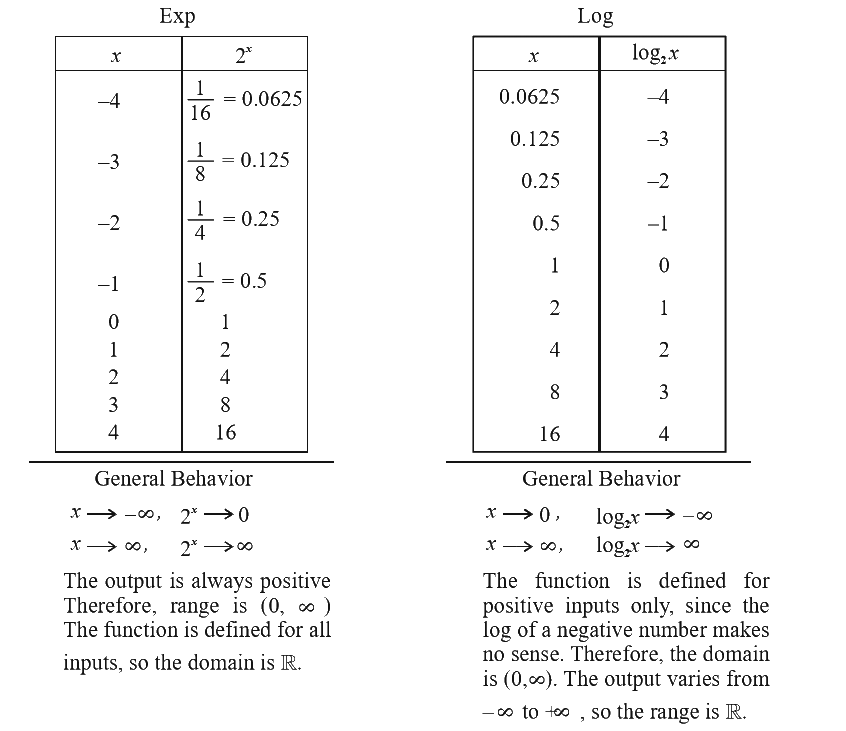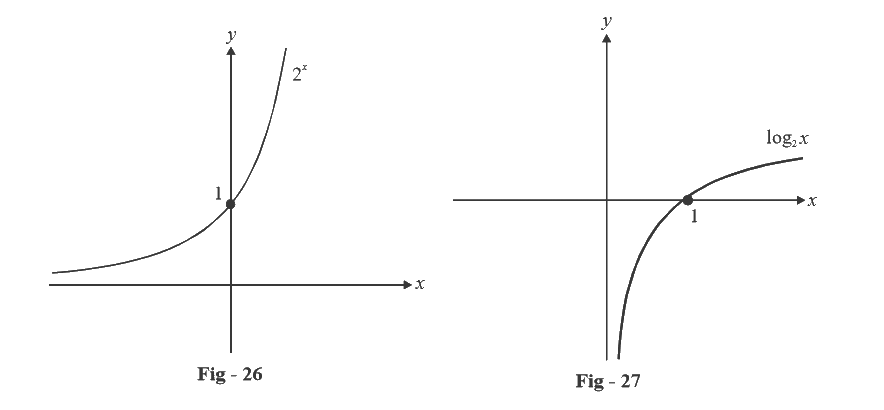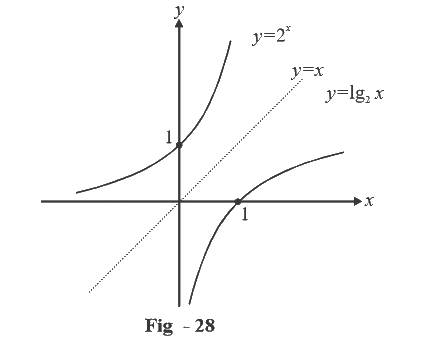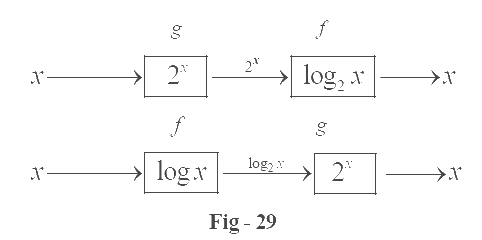# Introduction To Exponential and Logarithmic Functions

Go back to  'Functions'

In our experience beginning students are generally not very clear about the exponential and logarithmic functions. These are therefore being discussed in a dedicated section, and it is imperative that you take time in undeThereforerstanding these functions completely.

First of all, the outset itself, it is helpful to think of the exp. and log functions as inverses of each other. Examples:

$${2^3} = 8$$ is the same as saying that, $${\log_2}{\rm{ }}8{\rm{ }} = {\rm{ }}3$$

{3^{ - 4}} = \begin{align}\frac{1}{{81}}\end{align}is the same as saying that\begin{align} {\log _3}\left( {\frac{1}{{81}}} \right) = - 4\end{align}

In general,

$${a^b} = c$$ is equivalent to saying that $${\log _a}c = b$$

Now, we need to understand the exponentiation operation more carefully. We clearly understand the meaning of these terms.

\begin{align}&{2^3} = 2 \times 2 \times 2\\&{2^{ - 3}} = \frac{1}{{{2^3}}} = \frac{1}{2} \times \frac{1}{2} \times \frac{1}{2}\end{align}

Going further, terms like $${2^{1/2}}\;{\rm{and}}\;{3^{1/3}}$$ also make sense. $${2^{1/2}}$$ is real number which when squared will give 2. $${3^{1/3}}$$ is a real number which when cubed will give 3. In general, to any term of the form $${a^{p/q}}$$, we can attach the following interpretation:

${a^{p/q}} = {\left( {{a^{1/q}}} \right)^p} = {\left( {{q^{th}}\;{\rm{root}}\;{\rm{of}}\;a} \right)^p}$

So, we see that we can attach sence to any term of the form $${a^x}$$ where $$x$$ is rational, of the form \begin{align}\frac{p}{q}\end{align}. Additionally, we generally have $$a>0.$$ (Why should that be?)

Now, the following question arises: what if $$x$$ is irrational? What sence do we attach to $${a^x}$$ then? You example, how will we evaluate $$2\sqrt 2$$? Is this quantity defined? The answer is yes, but you have to be clear about how to interpret this quantity:

\begin{align}&\;\;\sqrt 2 = 1.41421......\\&\qquad = 1 + 0.4 + 0.01 + 0.004 + 0.0002 + 0.00001 + .......\\&\qquad = 1 + \frac{4}{{10}} + \frac{1}{{100}} + \frac{4}{{1000}} + \frac{2}{{10000}} + \frac{1}{{100000}} + .....\end{align}

This is how $$\sqrt 2$$ can be expressed as a sum of an infinite series of rationals. Now,

\begin{align}&{2^{\sqrt 2 }} \;\;= {2^{\left( {1 + \frac{4}{{10}} + \frac{1}{{100}} + \frac{4}{{1000}} + \frac{2}{{10000}} + \frac{1}{{100000}} + ....} \right)}}\\&\qquad = {2^1} \cdot {2^{\frac{4}{{10}}}} \cdot {2^{\frac{1}{{10}}}} \cdot {2^{\frac{4}{{1000}}}} \cdot {2^{\frac{2}{{10000}}}} \cdot {2^{\frac{1}{{100000}}}} \cdot ....\end{align}

Each of the terms in the product, as we’ve seen, has a well defined meaning, and therefore so does $${2^{\sqrt 2 }}$$. We can calculate $${2^{\sqrt 2 }}$$ as accurately as we wish by taking as many terms after the decimal point into account.

This discussion should make it clear that for $$a>0,$$ $${a^x}$$ is defined whether $$x$$ is positive or negative or zero, rational or irrational. If $$a$$ is a fixed constant and we let $$x$$ be a variable real number, we can define the general exponential function as

$f(x) = {a^x},\;\;x \in \mathbb{R}$

$$a$$ is called the base, and $$x$$ is the exponent. In terms of log, the same relation becomes

${\log _a}f(x) = x$

We will now understand the behaviour of the exp. and log functions in more detail by plotting their graphs. For that, it is necessary to get a numerical feel of their variation. We do this by calculating exp and log for a fixed base, say $$a=2,$$ and particular values of the variable $$x$$:Finally, let draw the graphs of the two function:Notice what happens if we draw $$f\left( x \right) = 2x{\rm{ }}\; and\; {\rm{ }}f\left( x \right) = {\rm{ }}{\log_2}x$$ on the same axis:

The graphs of the two functions seem to be mirror reflections of each other in the line $$y=x$$ (Infact, they are!)Also note what happens if we operate the  $$f\left( x \right) = {\log_2}x$$ function and then the $$g\left( x \right) = {\rm{ }}{2^x}$$ function on a particular $$x$$, in any order. (For the second sequence, $$x>0$$ so that $${\log_2}x$$ is defined.)That is $$f\,(g (x)) = x\;{\rm{and}}\;g\,(f\,(x)) = x.$$ Such functions are called inverse functions:

$$f = {g^{ - 1}}$$ or $$g = {f^{ - 1}}$$

The graphs of $$f$$ and $$g$$ are mirror reflections of each other about the line $$y=x.$$ (Note that because of this, the domain and range get interchanged)

We will study inverse functions in detail later.

For reasons that will become clear later in calculus, the base in exp/log functions is generally takes to be $$e.$$ What is $$e$$? Where did it come from? Why $$e$$ and not some other number? These questions will be answered over time. For now, it should be sufficient to know that $$e$$ is an irrational number with the approximate value 2.7182.

The graphs of $${e^x}$$ and $${\log_e}x$$ (denoted generally by $${l_{nx}}$$) will resemble the graphs of $${2^x}\;{\rm{and}}\;{\rm{lo}}{{\rm{g}}_2}x$$ respectively.